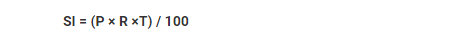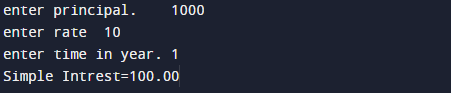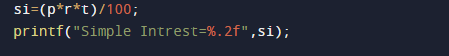c program to calculate simple interest

In this tutorial you will learn about the c program to calculate simple interest and its application with practical example.

In this tutorial, we will learn to create a C program that Find Simple Interest using C programming.

Prerequisites

Before starting with this tutorial we assume that you are best aware of the following C programming topics:

• Basic C programming.
• Operators.
• Basic input/output.
• Basic mathematics calculation.

What Is Simple interest ?

Simple interest is the interest amount given by the bank within a year to consumer or taken by bank from consumer  for a particular principal amount of money at some rate of interest. Simple Interest is a method of calculating the interest for a loan/principal amount.Where:=>
SI = simple interest.
P = principal
R = interest rate (in percentage).
T = time duration (in years).

OutputIn the above program, we have first declared and initialized a set variables required in the program.

• si = For calculating si.
• p=  for principal.
• r= for rate of interest.
• t= for time interval in a year.

First of all we declare 4 variable si,p,r and t then by prompting user to give principal rate and time . Here in this program we get principal rate and time from user.After accepting values of  principle, rate and time from user , we apply the formula to calculate simple interest is which  is si= (principle x rate x time) / 100. and  after calculating si we will print the Simple Interest.

Simple Interest is a method used by mostly in Banking and Economic sectors to calculate the interest charges on loans or in saving account by Bank or Economic Sectors.

In this tutorial we have learn about the c program to calculate simple interest and its application with practical example. I hope you will like this tutorial.